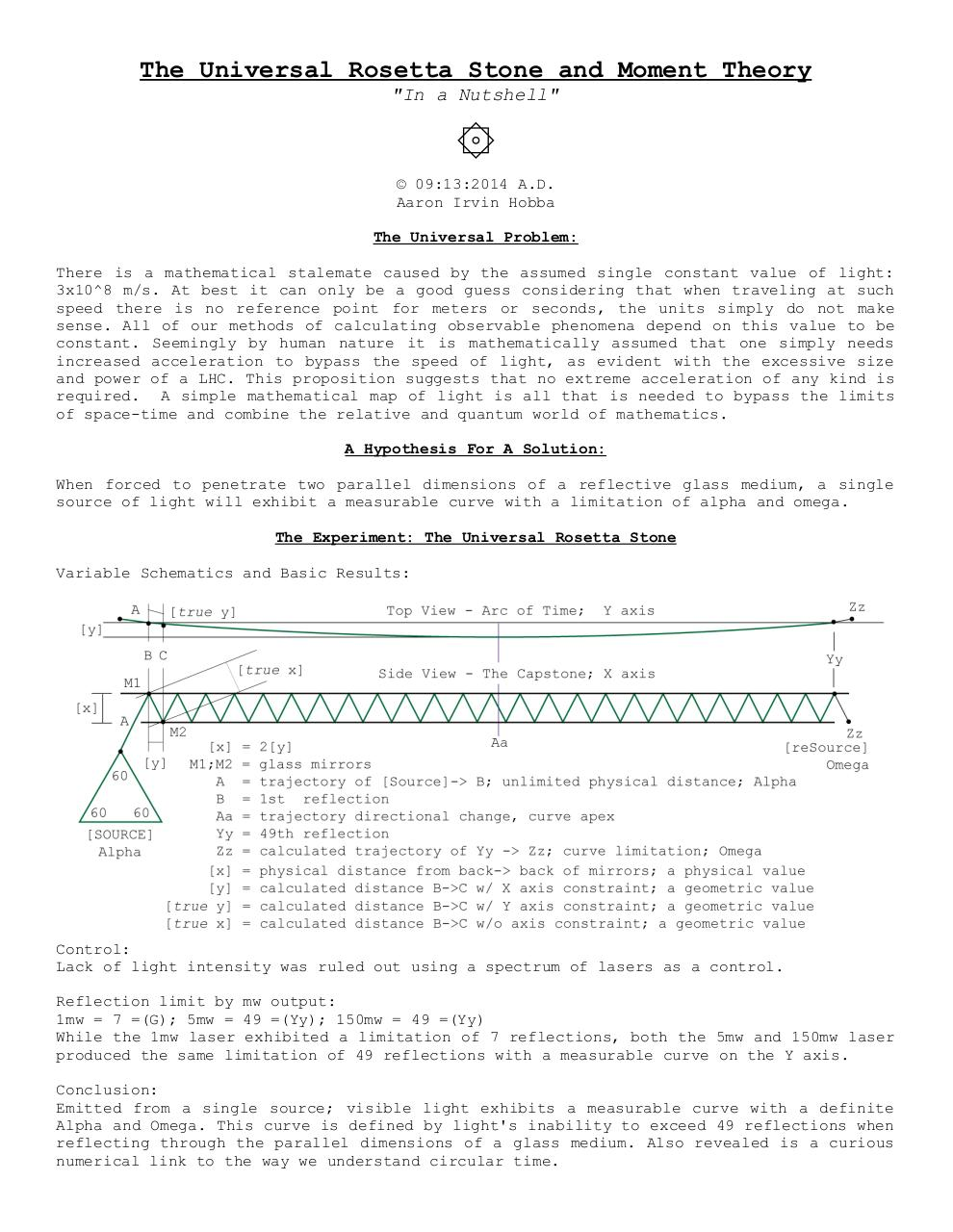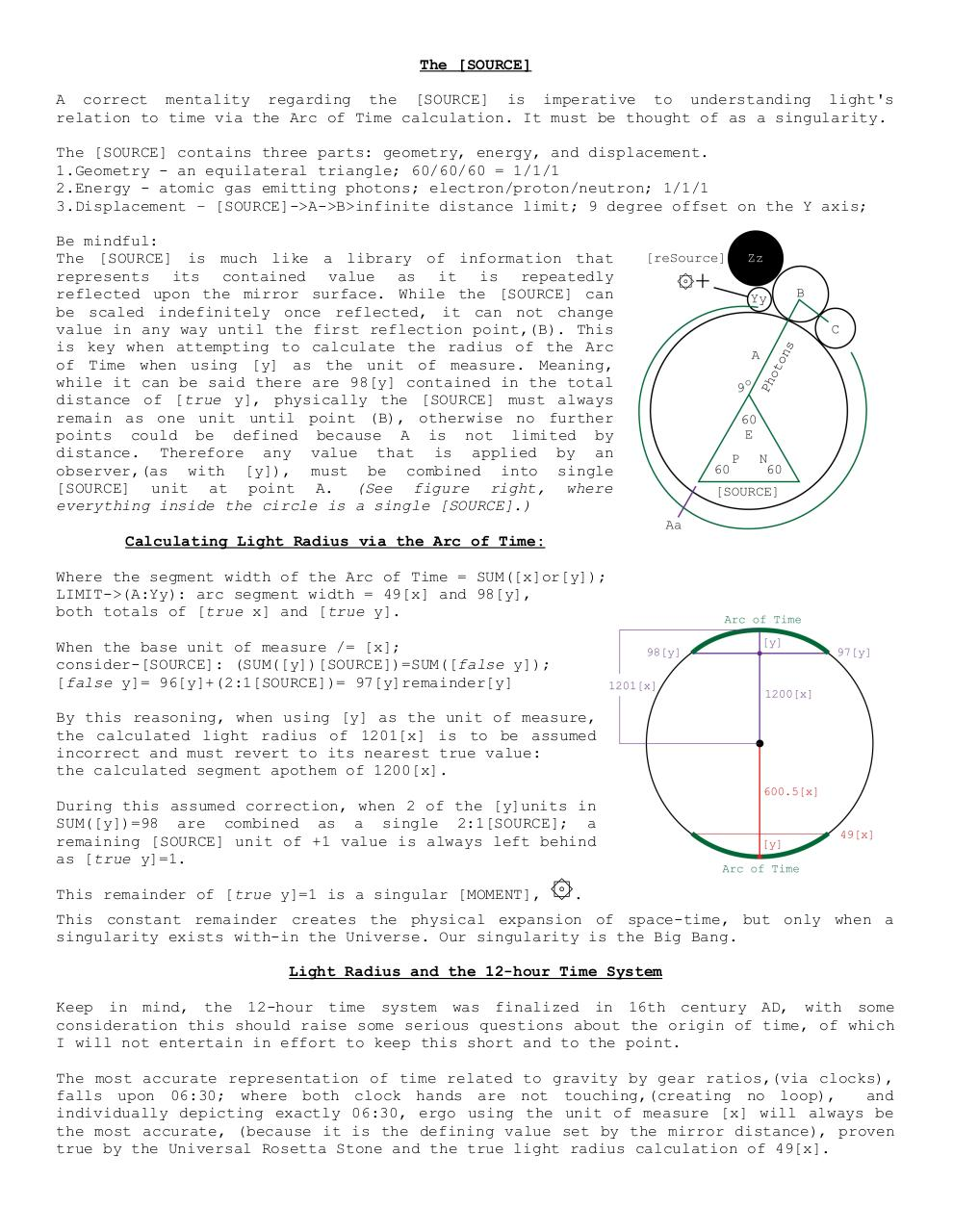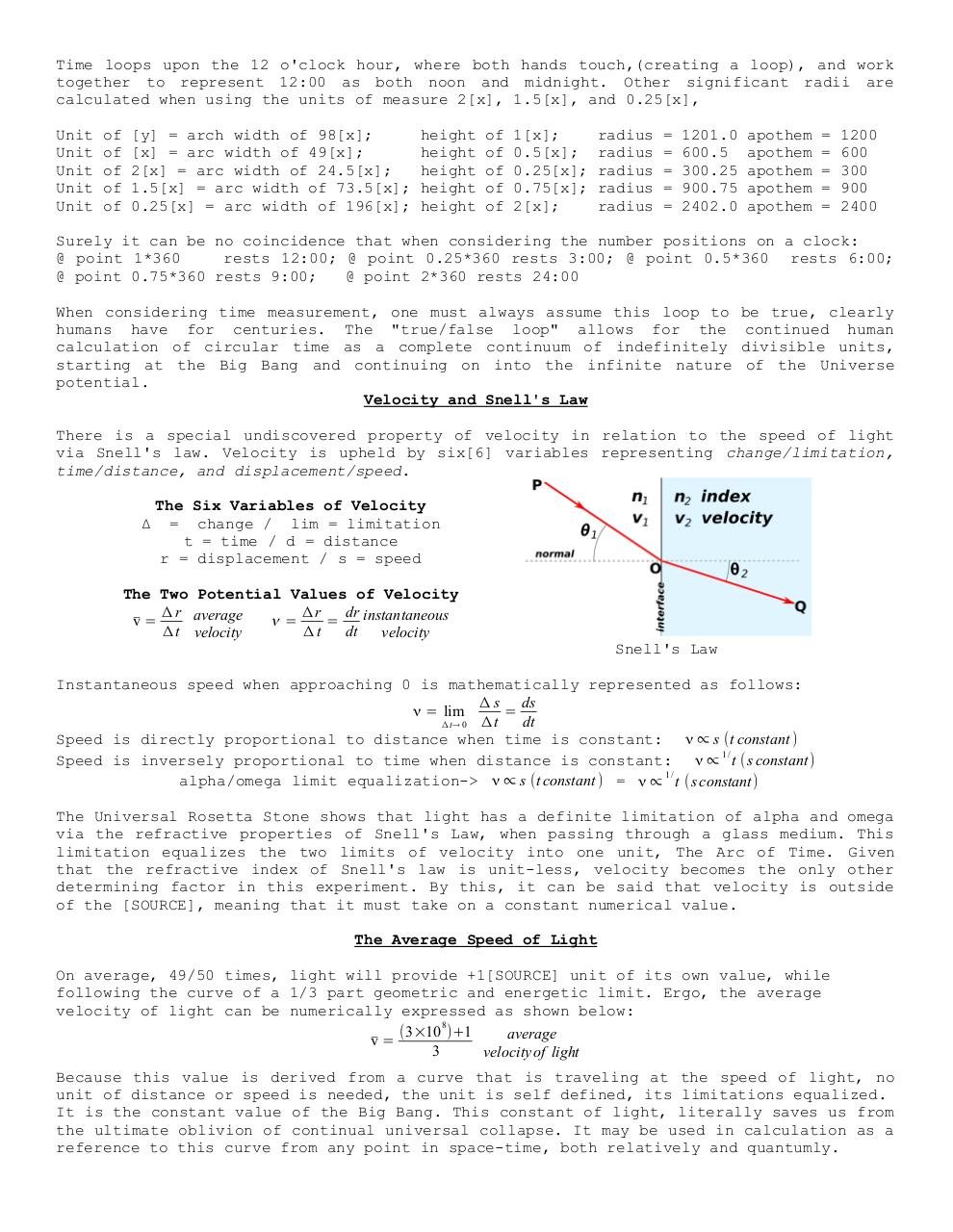# Moment Theory In A Nutshell .pdf

### File information

Original filename: Moment Theory In A Nutshell.pdf

This PDF 1.4 document has been generated by Writer / OpenOffice 4.1.1, and has been sent on pdf-archive.com on 20/01/2015 at 20:53, from IP address 174.59.x.x. The current document download page has been viewed 656 times.
File size: 471 KB (3 pages).
Privacy: public file

Moment Theory In A Nutshell.pdf (PDF, 471 KB)

### Document preview

The Universal Rosetta Stone and Moment Theory
"In a Nutshell"

۞

Aaron Irvin Hobba
The Universal Problem:
There is a mathematical stalemate caused by the assumed single constant value of light:
3x10^8 m/s. At best it can only be a good guess considering that when traveling at such
speed there is no reference point for meters or seconds, the units simply do not make
sense. All of our methods of calculating observable phenomena depend on this value to be
constant. Seemingly by human nature it is mathematically assumed that one simply needs
increased acceleration to bypass the speed of light, as evident with the excessive size
and power of a LHC. This proposition suggests that no extreme acceleration of any kind is
required. A simple mathematical map of light is all that is needed to bypass the limits
of space-time and combine the relative and quantum world of mathematics.
A Hypothesis For A Solution:
When forced to penetrate two parallel dimensions of a reflective glass medium, a single
source of light will exhibit a measurable curve with a limitation of alpha and omega.
The Experiment: The Universal Rosetta Stone
Variable Schematics and Basic Results:

Control:
Lack of light intensity was ruled out using a spectrum of lasers as a control.
Reflection limit by mw output:
1mw = 7 =(G); 5mw = 49 =(Yy); 150mw = 49 =(Yy)
While the 1mw laser exhibited a limitation of 7 reflections, both the 5mw and 150mw laser
produced the same limitation of 49 reflections with a measurable curve on the Y axis.
Conclusion:
Emitted from a single source; visible light exhibits a measurable curve with a definite
Alpha and Omega. This curve is defined by light's inability to exceed 49 reflections when
reflecting through the parallel dimensions of a glass medium. Also revealed is a curious
numerical link to the way we understand circular time.

The [SOURCE]
A correct mentality regarding the [SOURCE] is imperative to understanding light's
relation to time via the Arc of Time calculation. It must be thought of as a singularity.
The [SOURCE] contains three parts: geometry, energy, and displacement.
1.Geometry - an equilateral triangle; 60/60/60 = 1/1/1
2.Energy - atomic gas emitting photons; electron/proton/neutron; 1/1/1
3.Displacement – [SOURCE]-&gt;A-&gt;B&gt;infinite distance limit; 9 degree offset on the Y axis;
Be mindful:
The [SOURCE] is much like a library of information that
represents its contained value as it is repeatedly
reflected upon the mirror surface. While the [SOURCE] can
be scaled indefinitely once reflected, it can not change
value in any way until the first reflection point,(B). This
is key when attempting to calculate the radius of the Arc
of Time when using [y] as the unit of measure. Meaning,
while it can be said there are 98[y] contained in the total
distance of [true y], physically the [SOURCE] must always
remain as one unit until point (B), otherwise no further
points could be defined because A is not limited by
distance. Therefore any value that is applied by an
observer,(as with [y]), must be combined into single
[SOURCE] unit at point A. (See figure right, where
everything inside the circle is a single [SOURCE].)
Calculating Light Radius via the Arc of Time:
Where the segment width of the Arc of Time = SUM([x]or[y]);
LIMIT-&gt;(A:Yy): arc segment width = 49[x] and 98[y],
both totals of [true x] and [true y].
When the base unit of measure /= [x];
consider-[SOURCE]: (SUM([y])[SOURCE])=SUM([false y]);
[false y]= 96[y]+(2:1[SOURCE])= 97[y]remainder[y]
By this reasoning, when using [y] as the unit of measure,
the calculated light radius of 1201[x] is to be assumed
incorrect and must revert to its nearest true value:
the calculated segment apothem of 1200[x].
During this assumed correction, when 2 of the [y]units in
SUM([y])=98 are combined as a single 2:1[SOURCE]; a
remaining [SOURCE] unit of +1 value is always left behind
as [true y]=1.
This remainder of [true y]=1 is a singular [MOMENT],

۞.

This constant remainder creates the physical expansion of space-time, but only when a
singularity exists with-in the Universe. Our singularity is the Big Bang.
Light Radius and the 12-hour Time System
Keep in mind, the 12-hour time system was finalized in 16th century AD, with some
consideration this should raise some serious questions about the origin of time, of which
I will not entertain in effort to keep this short and to the point.
The most accurate representation of time related to gravity by gear ratios,(via clocks),
falls upon 06:30; where both clock hands are not touching,(creating no loop),
and
individually depicting exactly 06:30, ergo using the unit of measure [x] will always be
the most accurate, (because it is the defining value set by the mirror distance), proven
true by the Universal Rosetta Stone and the true light radius calculation of 49[x].

Time loops upon the 12 o'clock hour, where both hands touch,(creating a loop), and work
together to represent 12:00 as both noon and midnight. Other significant radii are
calculated when using the units of measure 2[x], 1.5[x], and 0.25[x],
Unit
Unit
Unit
Unit
Unit

of
of
of
of
of

[y] = arch width of 98[x];
[x] = arc width of 49[x];
2[x] = arc width of 24.5[x];
1.5[x] = arc width of 73.5[x];
0.25[x] = arc width of 196[x];

height
height
height
height
height

of
of
of
of
of

1[x];
0.5[x];
0.25[x];
0.75[x];
2[x];

=
=
=
=
=

1201.0
600.5
300.25
900.75
2402.0

apothem
apothem
apothem
apothem
apothem

=
=
=
=
=

1200
600
300
900
2400

Surely it can be no coincidence that when considering the number positions on a clock:
@ point 1*360
rests 12:00; @ point 0.25*360 rests 3:00; @ point 0.5*360 rests 6:00;
@ point 0.75*360 rests 9:00;
@ point 2*360 rests 24:00
When considering time measurement, one must always assume this loop to
humans have for centuries. The "true/false loop" allows for the
calculation of circular time as a complete continuum of indefinitely
starting at the Big Bang and continuing on into the infinite nature
potential.
Velocity and Snell's Law

be true, clearly
continued human
divisible units,
of the Universe

There is a special undiscovered property of velocity in relation to the speed of light
via Snell's law. Velocity is upheld by six variables representing change/limitation,
time/distance, and displacement/speed.
Δ

The Six Variables of Velocity
= change / lim = limitation
t = time / d = distance
r = displacement / s = speed

The Two Potential Values of Velocity
Δ r average
Δr
dr instantaneous
ν=
=
̄v =
Δ t velocity
Δt
dt
velocity
Snell's Law
Instantaneous speed when approaching 0 is mathematically represented as follows:
Δ s ds
ν = lim
=
dt
Δ t→ 0 Δ t
Speed is directly proportional to distance when time is constant: ν ∝ s (t constant )
1/
Speed is inversely proportional to time when distance is constant: ν ∝ t ( s constant)
1/
alpha/omega limit equalization-&gt; ν ∝ s (t constant ) = ν ∝ t ( s constant)
The Universal Rosetta Stone shows that light has a definite limitation of alpha and omega
via the refractive properties of Snell's Law, when passing through a glass medium. This
limitation equalizes the two limits of velocity into one unit, The Arc of Time. Given
that the refractive index of Snell's law is unit-less, velocity becomes the only other
determining factor in this experiment. By this, it can be said that velocity is outside
of the [SOURCE], meaning that it must take on a constant numerical value.
The Average Speed of Light
On average, 49/50 times, light will provide +1[SOURCE] unit of its own value, while
following the curve of a 1/3 part geometric and energetic limit. Ergo, the average
velocity of light can be numerically expressed as shown below:
8
(3×10 )+1
average
̄v =
3
velocity of light
Because this value is derived from a curve that is traveling at the speed of light, no
unit of distance or speed is needed, the unit is self defined, its limitations equalized.
It is the constant value of the Big Bang. This constant of light, literally saves us from
the ultimate oblivion of continual universal collapse. It may be used in calculation as a
reference to this curve from any point in space-time, both relatively and quantumly.#### HTML Code

Copy the following HTML code to share your document on a Website or Blog

#### QR Code### Related keywords